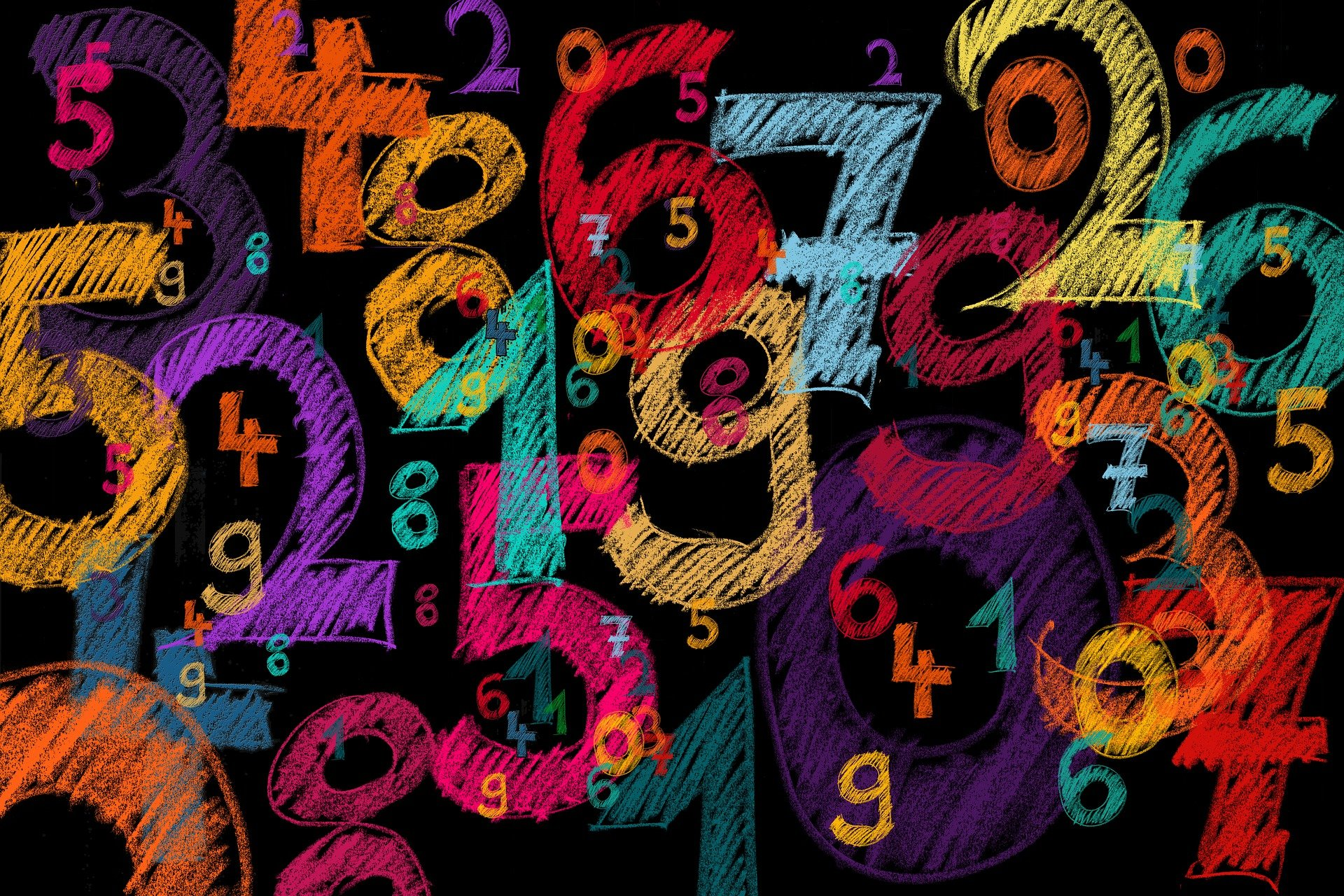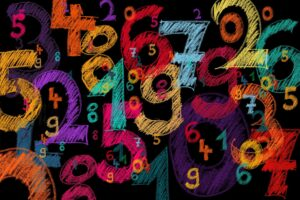# What is the Difference Between Factors and Multiples?What is the Difference Between Factors and Multiples? Factors and multiples are numbers in multiplication problems. Let’s take a look at a number — let’s use number 20.

Now let’s start with factors. If we are working with factors, we are going to call the number 20 our “product” (the answer to a multiplication problem). So, since we know the product, what are the factors of 20?

Factors are numbers that multiply together to get the product.

Here’s an easy one — 1 X 20 = 20.

So, we already know two factors of 20 — 1 and 20.

The numbers 4 and 5 are also factors of 20, right? 4 X 5 = 20

Can you think of any other factors of 20? Can any other numbers be multiplied together to get 20?

How about 2 X 10? Yep! 2 X 10 = 20.

Want to know another cool fact? All those numbers that get multiplied together are called “factor pairs.” 2 and 10 are a factor pair, 4 and 5 are a factor pair, and so on.

So, now let’s list all the factors of 20. They are 1,2,4,5,10, and 20.

What are MULTIPLES?

Multiples are all the products of a number. And unlike factors, there are an infinite number of multiples. Using the number 20 again, the multiples of 20 will be all the products of multiplying 20 by any number.

1 X 20 = 20, 2 X 20 = 40, 3 X 20 = 60…

You can also think of multiples as “skip counting.”

The multiples of 20 are 20, 40, 60, 80, 100, 120, 140, 160 — and they keep going and going.